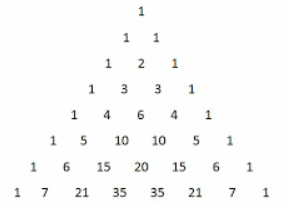# Pascal's triangle - a code with for-loops in Matlab

The Pascal's triangle is a triangular array of the binomial coefficients. The binomial coefficients appear as the numbers of Pascal's triangle. We can calculate the elements of this triangle by using simple iterations with Matlab. The idea is to practice our for-loops and use our logic.

In algebra, the binomial theorem describes the expansion of powers of a binomial. According to this theorem, it’s possible to expand the power (x + y)n into a sum involving terms of the form Axbyc, where the exponents b and c are positive integers with b + c = n, and the coefficient A of each term is an integer depending on n and b.

For example, the fourth row in the triangle shows numbers 1 3 3 1, and that means the expansion of a cubic binomial, which has four terms.

(x + y)3 = x3 + 3x2y + 3xy2 + y2

The rows of Pascal's triangle are enumerated starting with row r = 1 at the top. The numbers in each row are numbered beginning with column c = 1.

An easy construction of the triangle could be as follows...

- On row 1, write only the number 1.

- The first and last elements of every row are also 1. On row 2, we’ll write just 1 and 1.

- Then, to calculate the elements from row 3 on (other than the first and last figures), we’ll add the number from the previous row before our column and the number from the previous row in our column.

For example, the fifth row has these five terms (compare with the rows mentioned above)

1     4     6     4     1Let’s code that in Matlab...

function pt = pascal_triangle(n)

% The first two rows are constant
pt(1, 1) = 1;
pt(2, 1 : 2) = [1 1];

% If only two rows are requested, then exit
if n < 3

return
end

for r = 3 : n

% The first element of every row is always 1
pt(r, 1) = 1;

% Every element is the addition of the two elements
% on top of it. That means the previous row.
for c = 2 : r-1
pt(r, c) = pt(r-1, c-1) + pt(r-1, c);

end

% The last element of every row is always 1
pt(r, r) = 1;
end

We can try the function from the command window, just like this, with the number of desired rows as an input parameter:

pt = pascal_triangle(8)

and we get the 8-row matrix displaying the coefficients

pt =
1     0     0     0     0     0     0     0
1     1     0     0     0     0     0     0
1     2     1     0     0     0     0     0
1     3     3     1     0     0     0     0
1     4     6     4     1     0     0     0
1     5    10    10     5     1     0     0
1     6    15    20    15     6     1     0
1     7    21    35    35    21     7     1

The zeros appear naturally when we change the row in our algorithm.

From 'Pascals Triangle' to home

From 'Pascals Triangle' to Flow Control

 Top